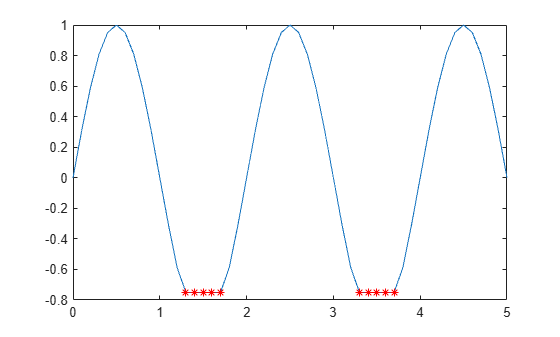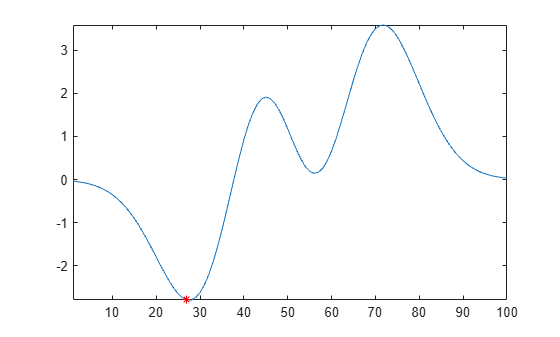# islocalmin

Find local minima

## Syntax

``TF = islocalmin(A)``
``TF = islocalmin(A,dim)``
``TF = islocalmin(___,Name,Value)``
``[TF,P] = islocalmin(___)``

## Description

example

````TF = islocalmin(A)` returns a logical array whose elements are 1 (`true`) when a local minimum is detected in the corresponding element of an array, table, or timetable.```

example

````TF = islocalmin(A,dim)` specifies the dimension of `A` to operate along. For example, `islocalmin(A,2)` finds local minima of each row of a matrix `A`.```

example

````TF = islocalmin(___,Name,Value)` specifies additional parameters for finding local minima using one or more name-value pair arguments. For example, `islocalmin(A,'SamplePoints',t)` finds local minima of `A` with respect to the time stamps contained in the time vector `t`.```

example

````[TF,P] = islocalmin(___)` also returns the prominence corresponding to each element of `A` for any of the previous syntaxes.```

## Examples

collapse all

Compute and plot the local minima of a vector of data.

```x = 1:100; A = (1-cos(2*pi*0.01*x)).*sin(2*pi*0.15*x); TF = islocalmin(A); plot(x,A,x(TF),A(TF),'r*')```Create a matrix of data, and compute the local minima for each row.

```A = -25*diag(ones(5,1)) + rand(5,5); TF = islocalmin(A,2)```
```TF = 5x5 logical array 0 0 0 1 0 0 1 0 0 0 0 0 1 0 0 0 0 0 1 0 0 0 1 0 0 ```

Compute the local minima of a vector of data relative to the time stamps in the vector `t`. Use the `'MinSeparation'` parameter to compute minima that are at least 45 minutes apart.

```t = hours(linspace(0,3,15)); A = [2 4 6 4 3 7 5 6 5 10 4 -1 -3 -2 0]; TF = islocalmin(A,'MinSeparation',minutes(45),'SamplePoints',t); plot(t,A,t(TF),A(TF),'r*')```Specify a method for indicating consecutive minima values.

Compute the local minima of data that contains consecutive minima values. Indicate the minimum of each flat region based on the first occurrence of that value.

```x = 0:0.1:5; A = max(-0.75, sin(pi*x)); TF1 = islocalmin(A, 'FlatSelection', 'first'); plot(x,A,x(TF1),A(TF1),'r*')```Indicate the minimum of each flat region with all occurrences of that value.

```TF2 = islocalmin(A, 'FlatSelection', 'all'); plot(x,A,x(TF2),A(TF2),'r*')```Compute the local minima of a vector of data and their prominence, and then plot them with the data.

```x = 1:100; A = peaks(100); A = A(50,:); [TF1,P] = islocalmin(A); P(TF1)```
```ans = 1×2 2.7585 1.7703 ```
```plot(x,A,x(TF1),A(TF1),'r*') axis tight```Compute the most prominent minimum in the data by specifying a minimum prominence requirement.

```TF2 = islocalmin(A,'MinProminence',2); plot(x,A,x(TF2),A(TF2),'r*') axis tight```## Input Arguments

collapse all

Input data, specified as a vector, matrix, multidimensional array, table, or timetable.

Data Types: `single` | `double` | `int8` | `int16` | `int32` | `int64` | `uint8` | `uint16` | `uint32` | `uint64` | `logical` | `table` | `timetable`

Operating dimension, specified as a positive integer scalar. By default, `islocalmin` operates along the first dimension whose size does not equal 1.

For example, if `A` is a matrix, then `islocalmin(A,1)` operates along the rows of `A`, computing local minima for each column.`islocalmin(A,2)` operates along the columns of `A`, computing local minima for each row.Data Types: `single` | `double` | `int8` | `int16` | `int32` | `int64` | `uint8` | `uint16` | `uint32` | `uint64`

### Name-Value Arguments

Specify optional comma-separated pairs of `Name,Value` arguments. `Name` is the argument name and `Value` is the corresponding value. `Name` must appear inside quotes. You can specify several name and value pair arguments in any order as `Name1,Value1,...,NameN,ValueN`.

Example: `TF = islocalmin(A,'MinProminence',2)`
Data Options

collapse all

Sample points, specified as the comma-separated pair consisting of `'SamplePoints'` and either a vector of sample point values or one of the options in the following table when the input data is a table. The sample points represent the x-axis locations of the data, and must be sorted and contain unique elements. Sample points do not need to be uniformly sampled. The vector `[1 2 3 ...]` is the default.

When the input data is a table, you can specify the sample points as a table variable using one of the following options.

Option for Table InputDescriptionExamples
Variable name

A character vector or scalar string specifying a single table variable name

`'Var1'`

`"Var1"`

Scalar variable index

A scalar table variable index

`3`

Logical vector

A logical vector whose elements each correspond to a table variable, where `true` specifies the corresponding variable as the sample points, and all other elements are `false`

`[true false false]`

Function handle

A function handle that takes a table variable as input and returns a logical scalar, which must be `true` for only one table variable

`@isnumeric`

`vartype` subscript

A table subscript generated by the `vartype` function that returns a subscript for only one variable

`vartype('numeric')`

Note

This name-value pair is not supported when the input data is a `timetable`. Timetables always use the vector of row times as the sample points. To use different sample points, you must edit the timetable so that the row times contain the desired sample points.

Example: `islocalmax(A,'SamplePoints',0:0.1:10)`

Example: `islocalmax(T,'SamplePoints',"Var1")`

Data Types: `double` | `single` | `int8` | `int16` | `int32` | `int64` | `uint8` | `uint16` | `uint32` | `uint64` | `datetime` | `duration`

Table variables to operate on, specified as the comma-separated pair consisting of `'DataVariables'` and one of the options in this table. The `'DataVariables'` value indicates which variables of the input table to examine for local minima. The data type associated with the indicated variables must be numeric or `logical`. Other variables in the table not specified by `'DataVariables'` are not operated on, so the output contains `false` values for those variables.

OptionDescriptionExamples
Variable name

A character vector or scalar string specifying a single table variable name

`'Var1'`

`"Var1"`

Vector of variable names

A cell array of character vectors or string array where each element is a table variable name

`{'Var1' 'Var2'}`

`["Var1" "Var2"]`

Scalar or vector of variable indices

A scalar or vector of table variable indices

`1`

`[1 3 5]`

Logical vector

A logical vector whose elements each correspond to a table variable, where `true` includes the corresponding variable and `false` excludes it

`[true false true]`

Function handle

A function handle that takes a table variable as input and returns a logical scalar

`@isnumeric`

`vartype` subscript

A table subscript generated by the `vartype` function

`vartype('numeric')`

Example: ```islocalmin(T,'DataVariables',["Var1" "Var2" "Var4"])```

Extrema Detection Options

collapse all

Minimum prominence, specified as the comma-separated pair consisting of `'MinProminence'` and a nonnegative scalar. `islocalmin` returns only local minima whose prominence is at least the value specified.

Data Types: `single` | `double` | `int8` | `int16` | `int32` | `int64` | `uint8` | `uint16` | `uint32` | `uint64`

Prominence window, specified as the comma-separated pair consisting of `'ProminenceWindow'` and a positive integer scalar, a two-element vector of positive integers, a positive duration scalar, or a two-element vector of positive durations. The value defines a window of neighboring points for which to compute the prominence for each local minimum.

When the window value is a positive integer scalar k, then the window is centered about each local minimum and contains k-1 neighboring elements. If k is even, then the window is centered about the current and previous elements. If a local minimum is within a flat region, then `islocalmin` treats the entire flat region as the center point of the window.

When the value is a two-element vector `[b f]` of positive integers, then the window contains the local minimum, `b` elements backward, and `f` elements forward. If a local minimum is within a flat region, then the window starts `b` elements before the first point of the region and ends `f` elements after the last point of the region.

When the input data is a timetable or when `'SamplePoints'` is specified as a `datetime` or `duration` vector, the window value must be of type `duration`, and the window is computed relative to the sample points.

Data Types: `single` | `double` | `int8` | `int16` | `int32` | `int64` | `uint8` | `uint16` | `uint32` | `uint64` | `duration`

Flat region indicator for when a local minimum value is repeated consecutively, specified as the comma-separated pair consisting of `'FlatSelection'` and one of the following:

• `'center'` — Indicate only the center element of a flat region as the local minimum. The element of `TF` corresponding to the center of the flat is 1, and is 0 for the remaining flat elements.

• `'first'` — Indicate only the first element of a flat region as the local minimum. The element of `TF` corresponding to the start of the flat is 1, and is 0 for the remaining flat elements.

• `'last'` — Indicate only the last element of a flat region as the local minimum. The element of `TF` corresponding to the end of the flat is 1, and is 0 for the remaining flat elements.

• `'all'` — Indicate all the elements of a flat region as the local minima. The elements of `TF` corresponding to all parts of the flat are 1.

When using the `'MinSeparation'` or `'MaxNumExtrema'` name-value pairs, flat region points are jointly considered a single minimum point.

Minimum separation between local minima, specified as the comma-separated pair consisting of `'MinSeparation'` and a nonnegative scalar. The separation value is defined in the same units as the sample points vector, which is ```[1 2 3 ...]``` by default. When the separation value is greater than 0, `islocalmin` selects the smallest local minimum and ignores all other local minima within the specified separation. This process is repeated until there are no more local minima detected.

When the sample points vector has type `datetime`, the separation value must have type `duration`.

Data Types: `single` | `double` | `int8` | `int16` | `int32` | `int64` | `uint8` | `uint16` | `uint32` | `uint64` | `duration`

Maximum number of minima detected, specified as the comma-separated pair consisting of `'MaxNumExtrema'` and a positive integer scalar. `islocalmin` finds no more than the specified number of most prominent minima, which is the length of the operating dimension by default.

Data Types: `single` | `double` | `int8` | `int16` | `int32` | `int64` | `uint8` | `uint16` | `uint32` | `uint64`

## Output Arguments

collapse all

Local minima indicator, returned as a vector, matrix, or multidimensional array. `TF` is the same size as `A`.

Data Types: `logical`

Prominence, returned as a vector, matrix, or multidimensional array. `P` is the same size as `A`.

If the input data has a signed or unsigned integer type, then `P` is an unsigned integer.

collapse all

### Prominence of Local Minimum

The prominence of a local minimum (or valley) measures how the valley stands out with respect to its depth and location relative to other valleys.

To measure the prominence of a valley, first extend a horizontal line from the valley to the left and to the right of the valley. Find where the line intersects the data on the left and on the right, which will either be another valley or the end of the data. Mark these locations as the outer endpoints of the left and right intervals. Next, find the highest peak in both the left and right intervals. Take the smaller of these two peaks, and measure the vertical distance from that peak to the valley. This distance is the prominence.

For a vector `x`, the largest prominence is at most `max(x)-min(x)`.